# C Program To Compute Nth Factorial Using Recursion

The program to compute factorial calls a function recursively to compute the output.The recursion is the ability of a computing procedure to call itself repeatedly until the problem is solved.

We compiled the program using Dev C++ version 5 (beta) compiler installed on Windows 7 64-bit system. You can use any standard C compiler, but make sure to change the source code according to compiler specifications.

You must know the following c programming concepts before trying the example program.

## Problem Definition

In simple words, the factorial of a given number n is the product of all the numbers up to that number, including the number.

So factorial of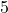is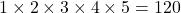In the previous section, we introduced you to the concept of recursion. To elaborate on that see the example below. If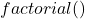is a function then,

Every time factorial function calls itself, it reduces 1 from the parameter of the function until the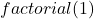is reached. Then it starts processing or working fromto the top –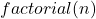and prints the final results.

## Program Code – Factorial with Recursion

/*Program to compute Nth factorial */
#include < stdio.h >
#include < stdlib.h >
int fact (int n)
{
unsigned int f;
if ((n == 0) || (n == 1))
return (n);
else
/* Compute factorial by Recursive calls */
f = n * fact (n - 1);
return (f);
}
main ()
{
int i, n;
printf ("Enter the Number :");
scanf ("%d", & n);
/* Printing results */
for (i = 0; i < 30; i++)
printf ("_");printf ("\n\n");
printf ("Factorial of Number %d is %d\n", n, fact (n));
for (i = 0; i < 30; i++)
printf ("_");printf ("\n\n");
system ("PAUSE");
return 0;
}

The most important line of code in the above source code is following.

f = n * fact(n-1);

The n is the current number being multiplied and function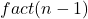calls the previous number and this process continues till 1 reached. Then, the actual multiplication starts from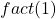up to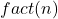processing all numbers and resulting in final output.

### Output

                Enter the Number:5
_______________________________
Factorial of Number 5 is 120
_______________________________